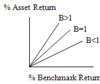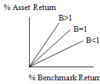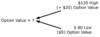Financial Management: Valuation Techniques Flashcards Preview

BEC > Financial Management: Valuation Techniques > Flashcards

Flashcards in Financial Management: Valuation Techniques Deck (35):
1

2

3

4

5

6

7

8

9

10

11

Plotting of CAPM:

The following graph shows the plotted slope of Beta under three assumptions as to its value:  A. When B = 1, a percentage change in an asset class benchmark return (i.e., a market) produces the same percentage change in an individual asset (e.g., a stock) of the same asset class. B. When B > 1, a percentage change in an asset class benchmark return produces a greater than equal change in an individual asset of the same asset class. C. When B < 1, a percentage change in an asset class benchmark return produces a less than equal change in an individual asset of the same asset class.12

13

14

Question:A graph that plots beta would show the relationship between :

Asset return and benchmark return:A graph which plots beta would show the relationship between the return of an individual asset and the return of the entire class of that asset, as reflected in a benchmark return for the class15

16

17

18

19

20

What is the Binomial Option Pricing Model (BOMP)?

Binomial Option Pricing Model: Another method for determining the value of an option. Uses "tree' diagram to estimate values at a number of time points between the valuation date and the expiration date.  It is more versatile than Black-Scholes Model. Binomial Option Pricing Model Steps: Generate price tree diagram--Use a branch for each desired period until expiration. Calculate option value at each tree end node as of the expiration date Calculate option value at each preceding node back to present valuation date. Example:  Assume an option for a share of stock that expires in one year and has an exercise price of \$100. An evaluation estimates that at the end of the year the underlying stock could have a price as high as \$120 and as low as \$80.  Assume that a .60 probability is assigned to the \$120 high value and a .40 probability (1.00 - .60) is assigned to the \$80 low value. The entity's cost of funds is 10%. Expected Value = [(.60 x \$20) + ( .40 x \$0)]/1.10 = [ \$12 + \$ 0 ]/1.10 Option Value = \$12/1.10 = \$10.9121

22

23

24

25

26

27

28

29

30

31

32

33

34

35